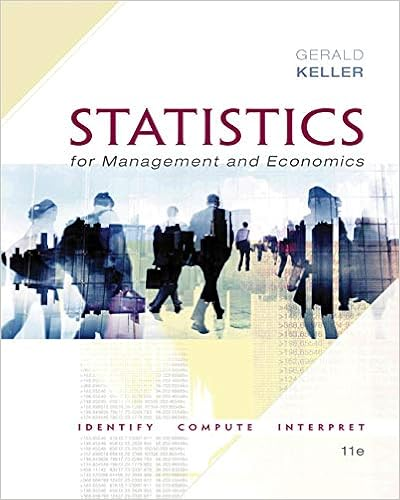# Judge the following sentence according to the

• Test Prep
• wildrider9896
• 182
• 75% (36) 27 out of 36 people found this document helpful

This preview shows page 51 - 53 out of 182 pages.

##### We have textbook solutions for you!
The document you are viewing contains questions related to this textbook.The document you are viewing contains questions related to this textbook.
Chapter 7 / Exercise 7.161
Statistics for Management and Economics + XLSTAT Bind-in
KellerExpert Verified
65) Judge the following sentence according to the criteria given below: Density decreases as temperature increases BECAUSE the molecules lose energy and slow down with increasing temperature. 65) _____ A) The assertion and the reason are both correct, and the reason is valid. B) The assertion and the reason are both correct, but the reason is invalid. C) The assertion is correct, but the reason is incorrect. D) The assertion is incorrect, but the reason is correct. E) Both the assertion and the reason are incorrect. 66) Judge the following sentence according to the criteria given below: Most of the physical properties of pure water and seawater are very similar BECAUSE seawater is 96.5% water. 66) _____ A) The assertion and the reason are both correct, and the reason is valid. B) The assertion and the reason are both correct, but the reason is invalid. C) The assertion is correct, but the reason is incorrect. D) The assertion is incorrect, but the reason is correct. E) Both the assertion and the reason are incorrect. 67) Which of the following processes causes sea surface salinity to increase near the Tropics of Cancer and Capricorn?67) _____ A) Freezing B) Evaporation C) Melting D) Polarization E) Condensation 68) Which of the following properties of pure water is different depending on the amount of water present? 68) _____ A) Density B) pH C) Odor D) Color E) Taste 69) Which of the following is the second most abundant negatively charged component of seawater? 69) _____ A) Calcium B) Chloride C) Magnesium D) Sodium E) Sulfate 70) Which of the following is the most abundant negatively charged component of seawater? 70) _____ A) Calcium B) Chloride C) Magnesium D) Sodium E) Sulfate 71) Which of the following is the second most abundant positively charged component of seawater? 71) _____ A) Calcium B) Chloride C) Magnesium D) Sodium E) Sulfate 72) Which of the following is the most abundant positively charged component of seawater? 72) _____ A) Calcium B) Chloride C) Magnesium D) Sodium E) Sulfate
##### We have textbook solutions for you!
The document you are viewing contains questions related to this textbook.The document you are viewing contains questions related to this textbook.
Chapter 7 / Exercise 7.161
Statistics for Management and Economics + XLSTAT Bind-in
KellerExpert Verified
73) Human consumption of which of the following components of seawater helps prevent goiter? 73) _____ A) Calcium B) Iodine C) Magnesium D) Sodium E) Sulfate 74) Which of the following hydrologic cycle reservoirs is the smallest? 74) _____ A) Streams and lakes B) World ocean C) Groundwater and soil moisture D) Atmospheric water vapor E) Ice caps, glaciers, and snow 75) On an annual basis which of the following fluxes between reservoirs of the hydrologic cycle is the largest? 75) _____ A) Atmosphere to ocean B) Continent to atmosphere C) Atmosphere to continent D) Ocean to atmosphere E) Continent to ocean
•••